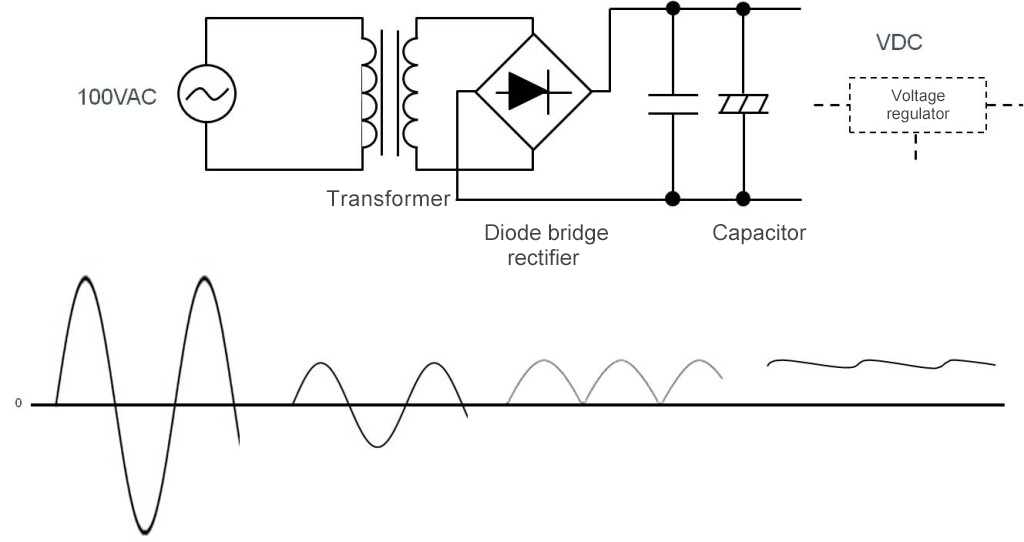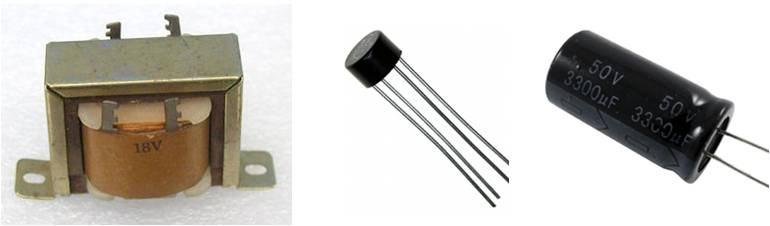Technical Information Site of Power Supply Design

2015.09.03 AC/DC

# Transformer System

AC/DC Basics

As a method of AC/DC conversion, we will explain a system that is based on a transformer. Figure 3 illustrates the general configuration of the transformer system.

Here we assume that the input voltage is 100VAC. The transformer steps down (transforms) the 100VAC input into an AC voltage from which a desired DC voltage can be produced. This part of operation constitutes an AC/AC conversion. The transformed level (the stepped down voltage generated on the secondary side of the transformer) is set in terms of a winding ratio between the primary and secondary sides of the transformer.Figure 3. Transformer based AC/DC conversion

If insulation is needed between the input and output sides, a transformer can be used to provide insulation.

The stepped-down AC voltage is converted to DC with a diode bridge rectifier, and smoothed out by a capacitor for final conversion to a DC voltage with small ripples. The rectified DC voltage is equal to the AC peak voltage (AC×√2) minus the forward voltage of the diode.

When regulation of output is not needed, the DC voltage can be used as an output. The initial value of the voltage depends upon the winding ratio in the transformer, and the voltage declines as the load current increases. If regulation is needed, it is performed using a voltage regulator. In this case, the voltage on the secondary side of the transformer must be set to a level suitable for conversion by the regulator.

If the ultimate goal is to create 12VDC, for example, a common approach is to set the voltage after rectification to approximately 18VDC so that it is not too low for proper operation and not too high to minimize losses.

Components used in a transformer system

The figure below shows examples of actual components used in a transformer based AC/DC conversion process.Figure 4. Example of components in transformer based AC/DC conversion. From the left: a transformer, a diode bridge rectifier, and an electrolytic capacitor

For the transformer used for AC/AC conversion, a low-frequency transformer is used since AC frequency is 50 or 60 Hz. Transformers that are designed as power supply units are called power transformers or commercial frequency transformers (commercial transformers). The size (volume) of a transformer may be thought of as being proportional to the output power of the power supply unit. A familiar example is AC adapters, which are supplied in units such that the larger their current capacity the bulkier and heavier the units. The basic structure of a transformer consists of an iron core, and primary and secondary winding wires. A core is commonly made of a silicon steel plate.

A diode bridge rectifier is supplied as a single package containing four diodes connected to perform rectifying. In terms of the shape of a diode bridge rectifier, in addition to the configuration shown in the photograph, there are SIP and DIP with a square package. Four single-unit rectifying diodes may be combined to form a diode bridge. The size of a diode also tends to become large as the amount of allowable current increases.

For a capacitor, basically an electrolytic capacitor is employed. The required capacitance, which varies with the load or allowable ripples, ranges from hundreds to thousands of μF. The larger the supply output power, the larger is also the size of the capacitor.

In a circuit that provides a supply voltage for general electronic circuits, the only component that handles high voltages is the transformer. For other components, a rated voltage matching the specific DC voltage to be supplied must be selected.

The transformer system has been in use most extensively for decades.

#### Key Points:

・The transformer system is most basic to the AC/DC conversion process.

・The output voltage just rectified is unregulated and it drops in proportion with increasing the load.